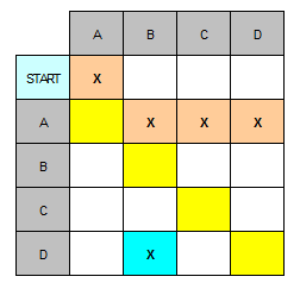# Manual methods – part 2 – precedence grid

### From the Work Breakdown Structure (WBS)

The use of this technique affords a step between the preparation of the work breakdown structure list and its conversion to a schedule. In terms of manual methods the next steps would be the preparation of Activity-on-Node networks and Activity-on-Arrow networks.

Once you have the work breakdown structure list you can build a precedence grid.
This grid serves to identify those tasks which must follow others.
When the first draft of the grid is completed it can be used to see potential errors and correct them.

Let us have a look at the above example based upon the simple list of tasks given below:

Task ‘A’ follows the START Task ‘B’ follows task ‘A’ Task ‘C’ follows task ‘A’ Task ‘D’ follows task ‘A’ Task ‘E’ follows task ‘C’ and ‘D’ Task ‘F’ follows task ‘E’ Task ‘G’ follows task ‘E’ Task ‘H’ follows task ‘G’ Task ‘I’ follows task ‘F’ and ‘H’ Task ‘END’ follows task ‘B’ and ‘I’

Note: There is no 'start' column in the following tasks and no 'end' row.
This is because nothing can come before the 'start' and nothing can happen after the 'end'.

Each column and row must have at least one ‘X’ in it. If there is one missing then the data needs to be checked.

If we look at all of the ‘X’s in a particular row they identify the tasks that must follow the task at the left end of the row.
For example, tasks ‘B’, ‘C’, and ‘D’ must follow task ‘A’.

It shows that these can start at the same time once task ‘A’ is complete.

The ‘X’s in a column show those tasks that go before the task at the top of the column.
For example, tasks ‘F’ and ‘H’ must go before task ‘I’.

The precedence grid is a ‘first draft’ from the work breakdown structure list that identifies the basic order of the tasks.
The grid above shows the crosses for the tasks listed above. On occasion you may have crosses in positions akin to the orange (F-E) and the blue (D-B) cells. These signify particular areas where you can eliminate a ‘cross’ as being redundant.

If we take a look at the orange cell (F-E) it is saying that task ‘E’ follows task ‘F’. This is OK but cell E-F says that task ‘F’ follows task ‘E’. They can’t both be true and we can eliminate the orange cell (F-E). We eliminate the later one as by our nomenclature ‘F’ should follow ‘E’ and not the other way round. That is, we assume that the basic order of carrying out the tasks has been agreed and would be task ‘A’ to task ‘I’ to complete the project.
Note that when you get cells that are a reflection about the middle diagonal (yellow squares) then redundant cells exist.

In a second case we see the blue cell at D-B. Notice that this is a particular case.
It is part of the system where multiple crosses in row ‘A’ coincide with the multiple crosses of column ‘B’.

In this case we have 3 crosses that form the corner of a square.
That is, cells (D-B), (A-B) and (A-D).
In the row for task ‘A’ we get task ‘B’ follows task ‘A’ and task ‘D’ follows task ‘A.’
These are seen more clearly in the smaller diagram below.So, both tasks ‘B’ and ‘D’ follow task ‘A’.
Also, we have task ‘B’ follows task ‘D’, cell (D-B).
This needs further examination.

We know from our work breakdown structure list and additional consideration that task ‘B’ will not start later than task ‘D’, hence we can remove cell (D-B). Of course, this may not be the case because our original work breakdown structure and task ordering may have been wrong. Seeing this anomaly allows us to think about the precedence.

### Uniqueness

When you produce your first Activity-on-Node or Activity-on-Arrow network you can visually compare that derived from the original work breakdown structure list and those derived from the precedence grid. If you produce an Activity-on-Node network from the original list and then try to compare it with the Activity-on-Node network from an updated version it is not easy to tell the difference quickly.

However, if you produce a precedence grid and review it, it is easier to see the alterations as you can lay one over the other.

There can be many precedence grids for each sub-project.